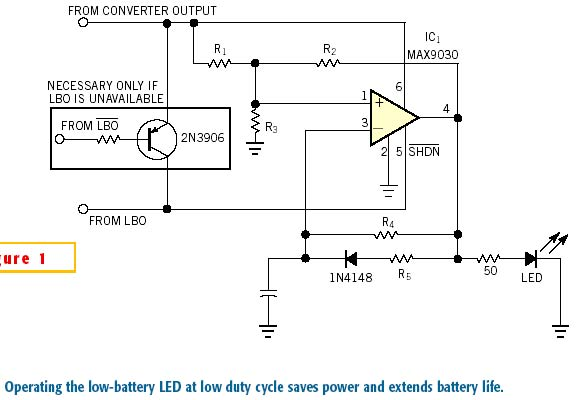# Low-Battery Indicator has High Efficiency

The usual method for implementing the low-battery warning featured in most battery-operated equipment is to illuminate an LED. However, the LED exacerbates the low-battery condition. You can greatly reduce the LED's power consumption by operating it at a low frequency and a low duty cycle.An existing LBO(low-battery output) like that found in dc/dc converters offers a convenient way to light the LED (Figure 1). IC1 is a small, inexpensive comparator with shutdown capability, housed in a six-pin SC70 package. It remains in shutdown condition while the battery is at normal operating levels but asserts LBO when the battery voltage falls below a preset threshold. Active-high LBO is usable as shown, but an active-low warning, , requires the optional circuitry shown. IC1 turns on, causing the LED to flash according to the following analysis: First, you want to keep the duty cycle low: DC=tON/(tON+tOFF).

You derive the on-time from the equation for time-varying voltage across a charging capacitor: V(t)=V(1–e–t/RC), so tON=–R5Cln(1–VTRIPHI/VOUT). You then derive the off-time from the equation for time-varying voltage across a discharging capacitor: V(t)=Ve–t/RC, so tOFF=–R4Cln(VTRIPLO/VOUT). Use Kirchoff's current laws to find the comparator's high and low trip levels: VTRIPHI=VOUT[R3(R1+R2)]/[R3(R1+ R2)+R1R2], and VTRIPLO=VOUT[R3R2]/[R3(R1+R2)+R1R2]. Assuming a 2.5% duty cycle and assuming that the LBO trips the comparator on when the battery voltage equals 3V, the resulting trip levels are 1V for low and 2V for high. The standard component values corresponding to this performance are: C1=0.1 µF, R1=R2=R3=1 MW , R4=3.6 MW , and R5=91 kW .

EDN

You may have to register before you can post comments and get full access to forum.
 User Name Remember Me? Password